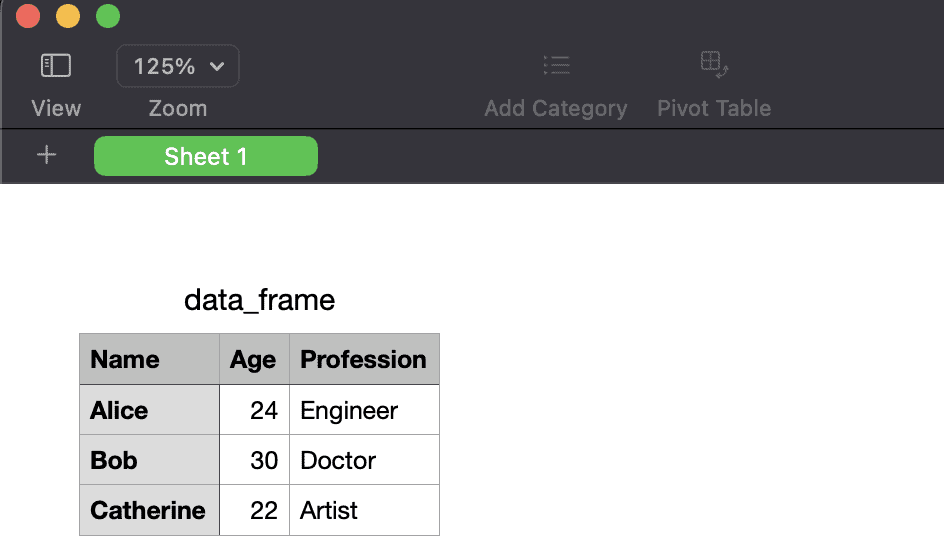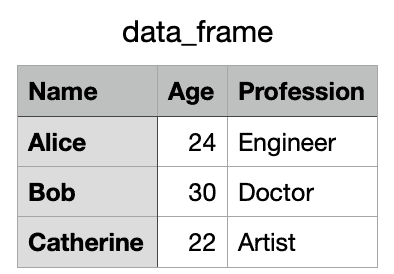# How to Export DataFrame to CSV in R

Here are three methods to export DataFrame to CSV in R:

1. Using write.csv() function from the base package
2. Using write_csv() function from readr package
3. Using fwrite() function from data.table package

## Method 1: Using write.csv() function

### Syntax

``````write.csv(x, file, traitsAsDir = FALSE, csv2 = TRUE,
row.names = FALSE, fileEncoding = "UTF-8" ...)``````

### Parameters

1. x: It is a data frame or other data added to the csv.
2. file: It is a character file name with or without a .csv extension, e.g., both “file” and “file.csv” are valid.
3. traitsAsDir: Logically, the result should be saved within trait folders; the construction is a file.path(dirname(), trait, basename()); folders are created if they do not exist.
4. csv2: It is a Logical export using write.csv2 or write.csv.
5. row.names: It is a Logical export row name as well.

### Example

``````df <- data.frame(
service_id = c(1:5),
service_name = c("Netflix", "Disney+", "HBOMAX", "Hulu", "Peacock"),
service_price = c(18, 10, 15, 7, 12),
stringsAsFactors = FALSE
)

write.csv(df, "shows.csv", row.names = FALSE)
cat("The data frame is exported", "\n")``````

Output

`` The data frame is exported``

And you can see in your current working directory that a file will be created called shows.csv.

### Exporting a CSV file without an index or row number

``````df <- data.frame(
Name = c("Juan", "Alcaraz", "Simantha"),
Age = c(22, 15, 19),
Vote = c(TRUE, FALSE, TRUE)
)

write.csv(df, file = "new_file.csv", row.names = FALSE)``````

Output

The newly created new_file.csv file has the following contents.

``````"Name","Age","Vote"
"Juan",22,TRUE
"Alcaraz",15,FALSE
"Simantha",19,TRUE``````

### Exporting a CSV file in which NA is replaced as an empty string

``````df <- data.frame(
Name = c("Juan", "Alcaraz", "Simantha"),
Age = c(22, 15, NA),
Vote = c(TRUE, NA, TRUE)
)

write.csv(df, file = "new_file.csv", na = "", row.names = FALSE)``````

Output

``````"Name","Age","Vote"
"Juan",22,TRUE
"Alcaraz",15,
"Simantha",,TRUE``````

You can see that we replaced NA values with “”(empty strings).

### Exporting a CSV file without quotes

``````df <- data.frame(
Name = c("Juan", "Alcaraz", "Simantha"),
Age = c(21, 19, 46),
Vote = c(TRUE, FALSE, TRUE)
)

write.csv(df, file = "new_file.csv", row.names = FALSE, quote = FALSE)``````

Output

``````Name,Age,Vote
Juan,21,TRUE
Alcaraz,19,FALSE
Simantha,46,TRUE``````

### Exporting a CSV file with encoding

``````df <- data.frame(
Name = c("Juan", "Alcaraz", "Simantha"),
Age = c(21, 19, 46),
Vote = c(TRUE, FALSE, TRUE)
)

write.csv(df, file = "new_file.csv", row.names = FALSE, fileEncoding = "UTF-8")``````

Output

``````Name,Age,Vote
Juan,21,TRUE
Alcaraz,19,FALSE
Simantha,46,TRUE``````

### Exporting CSV without Header using write.table()

``````df <- data.frame(
Name = c("Juan", "Alcaraz", "Simantha"),
Age = c(21, 19, 46),
Vote = c(TRUE, FALSE, TRUE)
)

write.table(df, file = "new_file.csv", col.names = FALSE)``````

Output

Check out the new_file.csv file. It has the following contents.

``````"1" "Juan" 21 TRUE
"2" "Alcaraz" 19 FALSE
"3" "Simantha" 46 TRUE``````

## Method 2: Using the write_csv() function from reader package

The write_csv() function from the readr package allows you to write a data frame to a CSV file easily. The function is designed to be a fast and flexible way to export data frames to CSV files.

If you haven’t installed the readr package yet, you can install it using:

``install.packages("readr")``

Here is the complete code example:

``````library(readr)

# Create a data frame
data_frame <- data.frame(
Name = c("Alice", "Bob", "Catherine"),
Age = c(24, 30, 22),
Profession = c("Engineer", "Doctor", "Artist")
)

# Write the data frame to a CSV file
write_csv(data_frame, "data_frame.csv")
``````

Output## Method 3: Using fwrite() function from data.table package

If you haven’t installed the data.table package yet, you can install it using:

``install.packages("data.table")``

Here’s how you can use the fwrite() function to export a data frame to a CSV file:

``````library(data.table)

# Create a data frame
data_frame <- data.frame(
Name = c("Alice", "Bob", "Catherine"),
Age = c(24, 30, 22),
Profession = c("Engineer", "Doctor", "Artist")
)

# Write the data frame to a CSV file
fwrite(data_frame, "data_frame.csv")
``````

Output## Related posts

1.